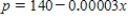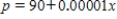# [Solved] Find the Equilibrium Point (X,p) of the Demand and Supply

Question 206
Multiple Choice

## Find the equilibrium point (x,p) of the demand and supply equations.The equilibrium point is the price p and number of units x that satisfy both the demand and supply equations. ​ Demand Supply​

A)(103, 1,250,000)
B)​(-1,250,000, -103)
C)​(1,250,000, 103)
D)​(103, -1,250,000)
E)​(-1,250,000, 103)

10+ million students use Quizplus to study and prepare for their homework, quizzes and exams through 20m+ questions in 300k quizzes.

### Mathematics

Explore our library and get Calculus Homework Help with various study sets and a huge amount of quizzes and questions

135

Study sets

2.1K

Quizzes

230.2K

Questions

Upload material to get free accessInvite a friend and get free accessSubscribe and get an instant access